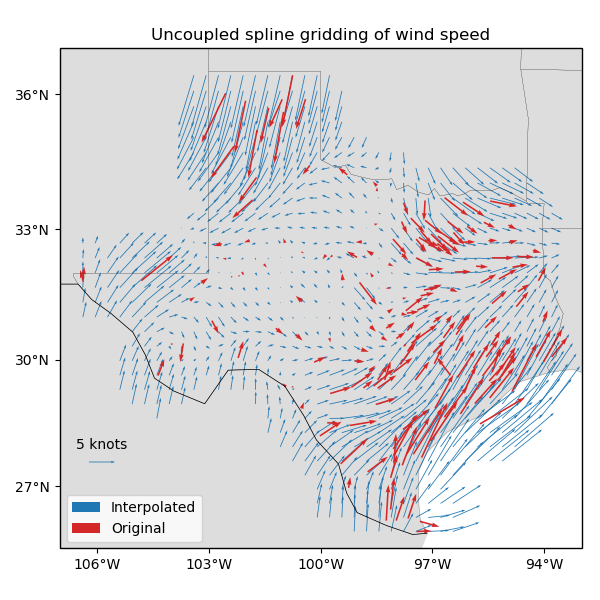# Gridding 2D vectors (uncoupled)¶

We can use verde.Vector to simultaneously process and grid all components of vector data. Each component is processed and gridded separately (see verde.VectorSpline2D for a coupled alternative) but we have the convenience of dealing with a single estimator. verde.Vector can be combined with verde.Trend, verde.Spline, and verde.Chain to create a full processing pipeline.Out:

station_id           ...            wind_speed_north_knots
0        0F2           ...                         -2.357185
1        11R           ...                          2.982564
2        2F5           ...                         -0.311412
3        3T5           ...                          3.018448
4        5C1           ...                          1.090743

[5 rows x 6 columns]
spacing=37000.0)), ('trend', Vector(components=[Trend(degree=1), Trend(degree=1)])), ('spline', Vector(components=[Spline(damping=1e-10, engine='auto', force_coords=None, mindist=500000.0), Spline(damping=1e-10, engine='auto', force_coords=None, mindist=500000.0)]))])
Cross-validation R^2 score: 0.78


import matplotlib.pyplot as plt
import cartopy.crs as ccrs
import numpy as np
import pyproj
import verde as vd

# Fetch the wind speed data from Texas.
data = vd.datasets.fetch_texas_wind()

# Separate out some of the data into utility variables
coordinates = (data.longitude.values, data.latitude.values)
region = vd.get_region(coordinates)
# Use a Mercator projection because Spline is a Cartesian gridder
projection = pyproj.Proj(proj="merc", lat_ts=data.latitude.mean())

# Split the data into a training and testing set. We'll fit the gridder on the training
# set and use the testing set to evaluate how well the gridder is performing.
train, test = vd.train_test_split(
projection(*coordinates),
(data.wind_speed_east_knots, data.wind_speed_north_knots),
random_state=2,
)

# We'll make a 20 arc-minute grid
spacing = 20 / 60

# Chain together a blocked mean to avoid aliasing, a polynomial trend (Spline usually
# requires de-trended data), and finally a Spline for each component. Notice that
# BlockReduce can work on multicomponent data without the use of Vector.
chain = vd.Chain(
[
("mean", vd.BlockReduce(np.mean, spacing * 111e3)),
("trend", vd.Vector([vd.Trend(degree=1) for i in range(2)])),
(
"spline",
vd.Vector([vd.Spline(damping=1e-10, mindist=500e3) for i in range(2)]),
),
]
)
print(chain)

# Fit on the training data
chain.fit(*train)
# And score on the testing data. The best possible score is 1, meaning a perfect
# prediction of the test data.
score = chain.score(*test)
print("Cross-validation R^2 score: {:.2f}".format(score))

# Interpolate the wind speed onto a regular geographic grid and mask the data that are
# far from the observation points
grid_full = chain.grid(
region, spacing=spacing, projection=projection, dims=["latitude", "longitude"]
)
coordinates, maxdist=3 * spacing * 111e3, grid=grid_full, projection=projection
)

# Make maps of the original and gridded wind speed
plt.figure(figsize=(6, 6))
ax = plt.axes(projection=ccrs.Mercator())
ax.set_title("Uncoupled spline gridding of wind speed")
tmp = ax.quiver(
grid.longitude.values,
grid.latitude.values,
grid.east_component.values,
grid.north_component.values,
width=0.0015,
scale=100,
color="tab:blue",
transform=ccrs.PlateCarree(),
label="Interpolated",
)
ax.quiver(
*coordinates,
data.wind_speed_east_knots.values,
data.wind_speed_north_knots.values,
width=0.003,
scale=100,
color="tab:red",
transform=ccrs.PlateCarree(),
label="Original",
)
ax.quiverkey(tmp, 0.17, 0.23, 5, label="5 knots", coordinates="figure")
ax.legend(loc="lower left")
# Use an utility function to add tick labels and land and ocean features to the map.
vd.datasets.setup_texas_wind_map(ax)
plt.tight_layout()
plt.show()


Total running time of the script: ( 0 minutes 0.627 seconds)

Gallery generated by Sphinx-Gallery